Problems & Puzzles: Puzzles

Puzzle 151.  Euler-Rodríguez set of consecutive primes

Luis Rodriguez post me two weeks ago the following nice puzzle:

Obtain the largest set of consecutive primes {p1, p2, p3, ... pk} such that:

P1 = P1 +0
p2 = p1+ 2
p3 = p2 +4
p4 = p3 +6
p5 = p4 +8
...
pk = pk-1 +2*(k-1)

Example for k=5: {347, 349, 353, 359, 367}

Maybe the skilled reader has already recognized that this pattern of primes is the same than the produced by the polynomial x2 + x + p1, for x=0, 1, 2, ... We already know (after Euler) that for p1 = 2, 3, 5, 11, 17 & 41, these polynomials are optimal in the sense that we get primes for all the values of 0<=x<=(p1-2). But we can not assure that all these prime values produced by the polynomial are consecutive.

The originality of the Rodríguez puzzle is to switch the question to the consecutiveness of the primes produced.

The largest example found by Luis was (k, p1) = (8, 128981)

By my own I found the following two least solutions for k=9 and k=10:

(9, 232728647) Luis Rodriguez found that the least is (9, 113575727)!
(10, 2426256797).

Questions

1. Find the least solutions for k>10
2. How large can be these kind of sets?

Solution:

Felice Russo sent (5/9/01) the following interesting argument to the question 2: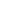Carlos

here below a heuristic argument on the question 2 of puzzle 151.

Let’s indicates with k the length of an Euler-Rodriguez (E-R) chain and with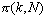the number of chains of length k up to N.

For N=10^j  with j=2,3,4,5,6,7,8 thanks to a Ubasic code I have found the following results: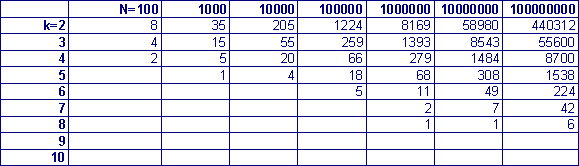Let’s  try now to found an asymptotic relationship for  the counting function.

According to a conjecture of M. Wolf  the probability that two consecutive primes  N and N+g have a gap g is given by: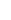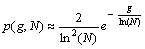In the E-R chains of length k we have exactly k-1 pairs of primes with gap 2,4,6,8,10….. and then the composite probability is given by: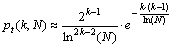So the counting function can be written as: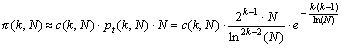(1)

For N large I have seen that c(k,N) is almost independent from N and a good approximation is given by: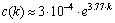(2)

Here below the plot of ratio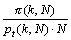versus k for N=10^8.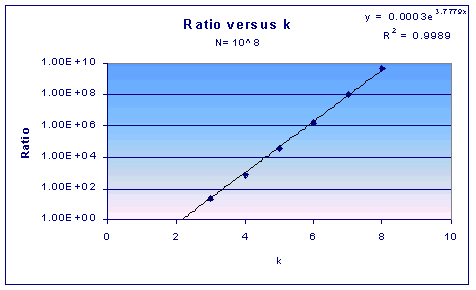By using the (1) and (2) the number of E-R chains for k between 2 and 10 and N from 100 to 10^8 has been calculated: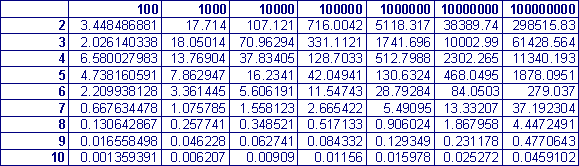The agreement with previous table is quite acceptable. Conclusion:

Since for any k :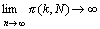the number of chains E-R for every given k is infinite. Moreover the length of the E-R chains can be as large as we want provided that N became large enough.

_____________________
 M. Wolf, Some conjectures on the gaps  between consecutive primes, preprint at :

***

The Felice Russo's argument is - probably - a specific case of the most general conjecture, the so-called "the k-tuple conjecture". This conjecture says in short that any admissible constellation of primes (and the studied one in this puzzle is an admissible constellation) occurs infinitely often.

Only one little shadow of doubt ness remains. Doest these arguments about the possibility of existence of E-R chains of any desired length contradict or not the "optimal" character of the polynomials x^2+x+q for q= 2, 3, 5, 11, 17 & 41 known to be the only to produce succesive (but not consecutive) primes when X goes from x=0 to x=q-2?

Who wants to explore this shadow?

***

I wrote to Felice Russo and told him "you are best suited to calculate what is the estimated value of p1 in order to happen the first ecample for k=40. Can you please do that?"

I assumed that we should detect a E-R chain of length k when the related counting function is around 1 and then putting N=10^j I obtained the following results for different k values:

k, j
9, 9
10, 10
11, 14
20, 42
30, 78
40, 118
50, 161
60, 206
80, 301

Then & according to this theory we may expect one E-R chain of 40 elements for p1 = 10^118.

***

Jean  Claude Rosa sent the following guide to make a faster search for the least example for k=12:

Let p1 prime, p1>7. If p1=1 mod 6 then p2=3 mod 6 so p2 is not prime

So 1) p1=6*n-1
If p1=0 mod 5 then p1, p5, p6, p10, p11 are not prime
p1=3 mod 5 then p2, p4, p7, p9, p12 are not prime
p1=5 mod 5 then p3, p8 are not prime

So 2) p1=5*n+1 ; 2
If p1=0 mod 7 then p1, p7, p8 are not prime
p1=1 mod 7 then p3, p5, p7, p10, p12 are not prime
p1=2 mod 7 then p4, p11 are not prime
p1=5 mod 7 then p2, p6, p9 are not prime

So 3) p1=7*n+3 ; 4 ; 6

And by combination of 1); 2); 3) we obtain :
p1=210*n+11 ; 17 ; 41 ; 101 ; 137 ; 167

By the same method with mod 11 we obtain (always for k=12)
p1=2310*n+17, 41, 221, 227, 347, 437, 521, 557, 587, 767, 851, 881, 941,
1007, 1151, 1217, 1271, 1277, 1361, 1427, 1481, 1511, 1607, 1691, 1907,
1931, 2141, 2201, 2237, 2267

***

Ken Wilke got two solutions (the earliest two?) for  11 consecutive primes, using the shortcut devised by Rosa. This is his email (23/10/2001):

I now have two verified solutions with 11 consecutive primes:

(A) 137376420947, 137376420949, 137376420953, 137376420959, 137376420967, 137376420977, 137376420989, 137376421003, 137376421019, 137376421037 and 137376421057; and

(B) 137168442221, 137168442223, 1371684472227, 137168442233, 137168442241, 137168442251,137168442263,137168442277, 13716844293, 137168442311 and 137168442331.

Clearly (B) is the smaller of the two.

Following Rosa's idea, i used the forms p1 = 210*n + 11;17;41;101;137 and
167. In my code, I let A = the product of the primes < 1000. Then to
shorten some of the checking of primes with the generated possible primes,
I used a gcd comparison with the product of primes < 1000. Also I used a
Fermat Theorem test to sieve out primes in the intervals between the
generated possible primes.

After checking the gcd, I instituted a second sieve based on the fact that
3 is a quadratic residue of all primes of the form 12n + 1  and a quadratic
non-residue of the primes of form 12n + 5. We denote a quadratic residue as
R and a quadratic non-residue as N. To illustrate with the form 210k + 11,
note that the string of desired primes is 210k + 11, 210k + 13, 210k + 17,
210 k + 23, 210k + 31, 210k + 41, 210k + 53, 210k + 67, 210k + 83, 210k +
101 and 210k + 121. Now if the first prime, 30k + 11, has an even value of
k, then the string can be represented as R, R, N, R, N, N, N, N, R, N, R.
If k is odd the sequence if quadratic residues is N, N, R, N, R, R, R, R,
N, R, N.

For each of the other forms we proceed similarly; however, in each case the
found taken in the appropriate order.

After this sieve, I used a "Fermat Theorem" test to verify the primality of
each prime in the sequence. Then I used several other "Fermat Theorem"
tests to sieve out primes in the intervals between the generated primes.

IMPORTANT NOTE: KEN HAS SENT TO ME HIS CODES (Ubasic) TO BE DISTRIBUTED FREELY TO THE PUZZLERS INTERESTED IN THEM, ON REQUEST.

***

At this very moment(23/10/2001) the results (n=5 to 11) can be summarized in the following table:

 n A Autor 5 347 L.R 6 13901 L.R 7 665111 L.R 8 128981 L.R 9 113575727 L.R 10 2426256797 C.R 11 137168442221 Wilke 12 4,656,625,081,181. J. K Andersen (12/2/03) 13 101,951,758,179,851. J. K Andersen (12/2/03) 14 484,511,389,338,941. J. K Andersen (12/2/03)

BTW, Luis Rodríguez notices that "hay una cosa que me llama la atención y es lo pronto que han aparecido los grupos de n primos consecutivos. Russo había predicho 10^14 para n = 11 y ahora resulta que aparece en 10^11. El grupo de 8 en 128981,tambien está anormalmente bajo.".

Does the Russo's theoretical approach needs a revision?

***

J. K. Andersen wrote (12/2/03):

I worked on this after a request from Luis Rodríguez.

All my results are based on primes proved with Tony Forbes' VFYPR, not just prp's. Note that elimination of a smaller potential solution with k primes requires a primality proof of an intermediate number to be sure that the primes are not consecutive.

My proven tests shows that Ken Wilke found the two smallest solutions for k=11. I found these additional minimal solutions:

k=12: A = 4,656,625,081,181.
k=13: A = 101,951,758,179,851.
k=14: A = 484,511,389,338,941.

The minimal solution for k=15 is above 15*10^15. The closest I got is A = 8,002,271,580,759,497. A+104 is the only intermediate prime.

The solutions agree badly with Felice Russo's estimates. I don't know the precision of his starting formula, but his calculations assumes the gaps have independent probabilities. I find strong dependencies in divisibility by small primes. The following assumes p1>100.

If p1 is prime (not divided by 2) then 2 never divides x^2+x+p1. If p1,p1+2 are primes (not divided by 3) then 3 never divides x^2+x+p1. If p1,p1+2,p1+6 are primes (not divided by 5) then 5 never divides x^2+x+p1.

There are several similar rules and they seem to increase the chance of getting more primes in a sequence starting with primes. Then the probability of a k-tuple becomes higher and the expected minimal solution becomes smaller. I have not made my own estimates of the number of solutions but I also expect infinitely many for all k.

The solutions were found with a modified version of the C program I wrote for puzzle 206. It only trial factors cases where none of the n numbers have a factor<=31. The surviving candidates are prp-tested with PrimeForm/GW but the time for this part is insignificant.

I stopped my search for k=15 at A=10^17 without finding a solution.

***Records   |  Conjectures  |  Problems  |  PuzzlesHome | Melancholia | Problems & Puzzles | References | News | Personal Page | Puzzlers | Search | Bulletin Board | Chat | Random Link Copyright © 1999-2012 primepuzzles.net. All rights reserved.